• 二叉树后序遍历的规律：左右根 后序非递归遍历中，访问根（子根）结点有两种情况 ①：遍历完左子树，需要遍历右子树，需要从栈中访问最顶上的根（子根）结点从而得到右子树的指针。 ②遍历完右子树，需要访问根（子...
二叉树非递归后序遍历算法(C语言)
二叉树后序遍历的规律：左右根
后序非递归遍历中，访问根（子根）结点有两种情况
①：遍历完左子树，需要遍历右子树，需要从栈中访问最顶上的根（子根）结点从而得到右子树的指针。
②遍历完右子树，需要访问根（子根）结点。
基于这个特性，我们需要区分，到底这个根结点，是访问完了左子树还是右子树，所以需要添加一个变量帮助辨认。
void Postorder(BiTree T){
Stack S;  //用于记录根和字根结点
InitStack(S);//初始化栈
BiNode *p=T;//临时变量记录记录当前访问的结点
BiNode *r = NULL; //临时变量，记录上一个访问到的结点，因为从右边访问根结点必定是右子树已经遍历完了，此时上一个访问的结点必定是右子树的根结点
while(p||!IsEmpty(S){
if (p!=NULL){
push(S,p);
p = p->lchild;
}else{
GetTop(S,p);//只读取根结点，不对栈内结点进行操作
//没有对右子树进行操作过
if(p->rchild!=NULL&&p->rchild!=r){
p=p->rchild;
push(S,p);
p=p->lchild;
}else{
pop(S,p);
visit(p);//对p进行访问，可以进行打印等操作
r=p;//记录当前访问的是p结点
p==NULL;//把p置空，进入下一次循环，直到栈内无元素，且p为空时遍历完成
}//else
}//else
}//while
}

代码讲解：https://www.bilibili.com/video/BV1it4y1X7xd/


展开全文算法 数据结构
• 五分钟C语言实现常见数据结构今天的内容分享的是二叉树后序遍历 DP问题，欢迎关注 动态规划一篇就够了 全网最详细, 逐步理解, 万字总结 - Johngo的文章 - 知乎 https://zhuanlan.zhihu.com/p/130743652二叉树后序...
五分钟C语言实现常见数据结构今天的内容分享的是二叉树后序遍历     DP问题，欢迎关注    动态规划一篇就够了 全网最详细, 逐步理解, 万字总结 - Johngo的文章 - 知乎 https://zhuanlan.zhihu.com/p/130743652二叉树后序遍历后序遍历过程递归实现非递归实现二叉树后序遍历二叉树的遍历方式主要由先序遍历、中序遍历和后续遍历，还后就是层次遍历感受完前两篇的遍历方式，本节来看看后序遍历后序遍历过程a. 先序遍历其左子树；b. 先序遍历其右子树；c. 访问根节点；然后就是一直递归下去，在访问到节点的时候，可以进行节点的相关处理，比如说简单的访问节点值下图是一棵二叉树，我们来手动模拟一下后序遍历过程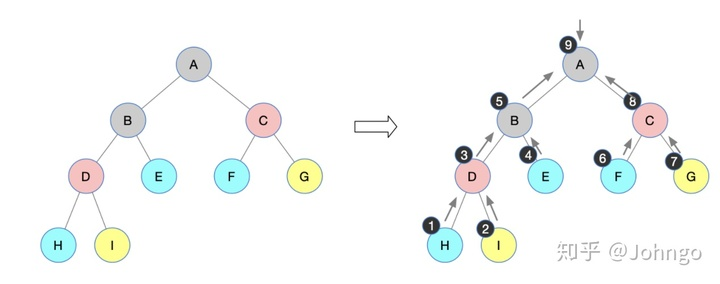按照上述后序遍历的过程，得到后序遍历序列：H I D E B F G C A递归实现二叉树的后序遍历利用上述的递归思想进行C语言代码实现：树形结构按照上述树形结构进行初始化# include <stdio.h>
# include <string.h>
# include <stdlib.h>
# define ElementType char

//结点结构体
typedef struct BinTNode{
ElementType data;
struct BinTNode * left;
struct BinTNode * right;
}BinTNode, *BinTree;

// 初始化树形结构
BinTNode * CreateBiTree(BinTNode *T){
T=(BinTNode*)malloc(sizeof(BinTNode));
T->data='A';
T->left=(BinTNode*)malloc(sizeof(BinTNode));
T->left->data='B';
T->right=(BinTNode*)malloc(sizeof(BinTNode));
T->right->data='C';

T->left->left=(BinTNode*)malloc(sizeof(BinTNode));
T->left->left->data='D';
T->left->right=(BinTNode*)malloc(sizeof(BinTNode));
T->left->right->data='E';
T->left->right->left=NULL;
T->left->right->right=NULL;
T->left->left->left=(BinTNode*)malloc(sizeof(BinTNode));
T->left->left->left->data='H';
T->left->left->left->left=NULL;
T->left->left->left->right=NULL;
T->left->left->right=(BinTNode*)malloc(sizeof(BinTNode));
T->left->left->right->data='I';
T->left->left->right->left=NULL;
T->left->left->right->right=NULL;

T->right->left=(BinTNode*)malloc(sizeof(BinTNode));
T->right->left->data='F';
T->right->left->left=NULL;
T->right->left->right=NULL;
T->right->right=(BinTNode*)malloc(sizeof(BinTNode));
T->right->right->data='G';
T->right->right->left=NULL;
T->right->right->right=NULL;

return T;
}

// 遍历过程中，输出节点值
void printElement(BinTNode * T){
printf("%c ",T->data);
}

//先序遍历
void PostOrderTraverse(BinTNode * T){
if (T) {
PostOrderTraverse(T->left);  //递归访问左孩子
PostOrderTraverse(T->right); //递归访问右孩子
printElement(T);             //输出节点值
}
// 当节点为空的时候，返回
return;
}

int main() {
BinTNode * Tree;
Tree = CreateBiTree(Tree);	// 初始化树形结构
printf("后序遍历: ");
PostOrderTraverse(Tree); 	// 先序递归进行
printf("n");   		// 最后换行
return 0;
}运行结果：后序遍历: H I D E B F G C A非递归实现相比于之前的先序遍历和中序遍历非递归实现，后序遍历的非递归算法与之前有所不同，后续遍历需要先访问左右子结点后，才能访问该结点，而这也是非递归的难点所在。考虑了几种实现的方案，这里我给出我认为比较清晰的一个方案供大家参考：借助两个栈（S1和S2）来进行操作看下面伪代码： 初始化S1的top元素是树的根结点;
while(top1 != -1) {
将栈顶元素push到S2;
if(栈顶元素有孩子结点){
按照孩子结点的左右顺序push进S1;
}
}
循环逐个弹出S2的栈顶元素伪代码可能看了之后有些不太好懂，但是还算看着清晰吧，下面借助图，一定会思路清晰的（长图发放）：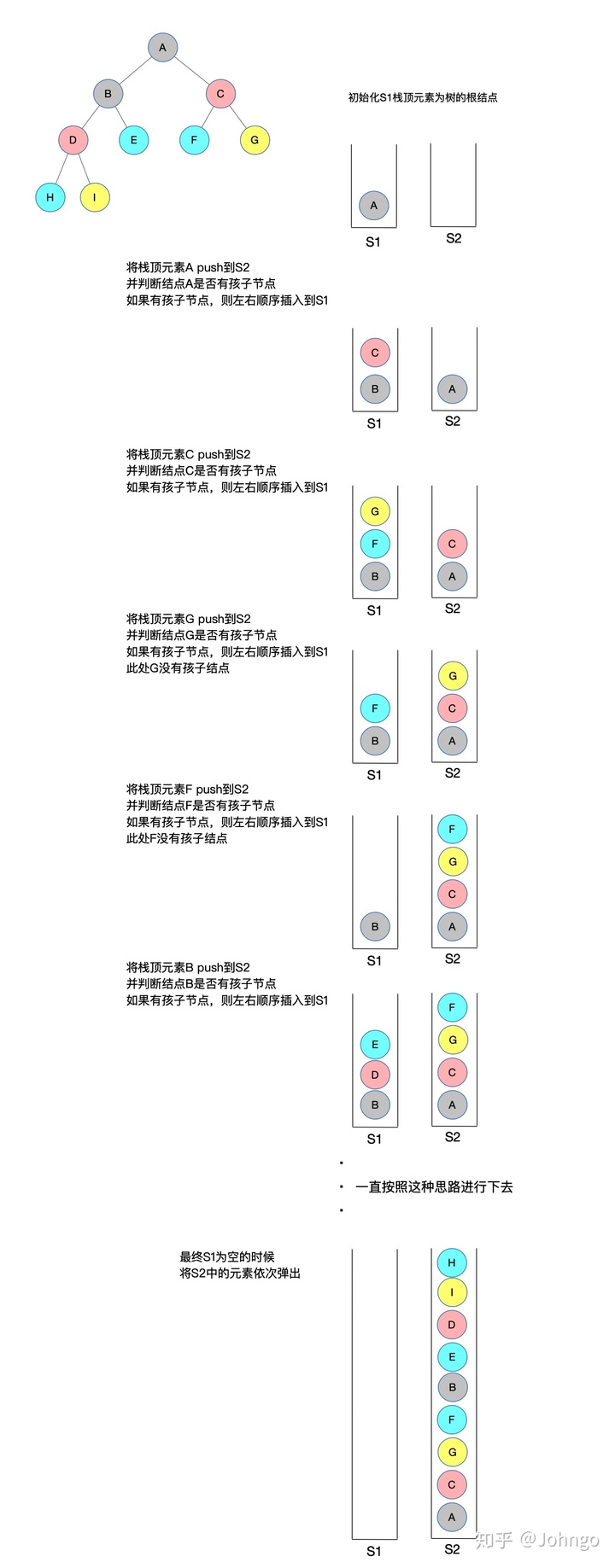有了这个思路就应该会很清晰了，下面按照上述思路用C语言实现：#include <stdio.h>
#include <string.h>
#include <stdlib.h>
#define ElementType char
int top_S1 = -1;   //定义栈S1 top下标
int top_S2 = -1;   //定义栈S2 top下标

// 结点结构体
typedef struct BinTNode{
ElementType data;
struct BinTNode * left;
struct BinTNode * right;
}BinTNode, *BinTree;

// 初始化树形结构
BinTNode * CreateBiTree(BinTNode *T) {
T=(BinTNode*)malloc(sizeof(BinTNode));
T->data='A';
T->left=(BinTNode*)malloc(sizeof(BinTNode));
T->left->data='B';
T->right=(BinTNode*)malloc(sizeof(BinTNode));
T->right->data='C';

T->left->left=(BinTNode*)malloc(sizeof(BinTNode));
T->left->left->data='D';
T->left->right=(BinTNode*)malloc(sizeof(BinTNode));
T->left->right->data='E';
T->left->right->left=NULL;
T->left->right->right=NULL;
T->left->left->left=(BinTNode*)malloc(sizeof(BinTNode));
T->left->left->left->data='H';
T->left->left->left->left=NULL;
T->left->left->left->right=NULL;
T->left->left->right=(BinTNode*)malloc(sizeof(BinTNode));
T->left->left->right->data='I';
T->left->left->right->left=NULL;
T->left->left->right->right=NULL;

T->right->left=(BinTNode*)malloc(sizeof(BinTNode));
T->right->left->data='F';
T->right->left->left=NULL;
T->right->left->right=NULL;
T->right->right=(BinTNode*)malloc(sizeof(BinTNode));
T->right->right->data='G';
T->right->right->left=NULL;
T->right->right->right=NULL;

return T;
}

// 栈S1 - 进栈push
void push_S1(BinTNode** stack,BinTNode * elem) {
stack[++top_S1] = elem;
}

// 栈S2 - 进栈push
void push_S2(BinTNode** stack,BinTNode * elem) {
stack[++top_S2] = elem;
}

//栈S1 - 弹栈pop
void pop_S1(){
if (top_S1 == -1) {
return ;
}
top_S1--;
}

//栈S2 - 弹栈pop
void pop_S2(){
if (top_S2 == -1) {
return ;
}
top_S2--;
}

// 遍历过程中，输出结点值
void printElement(BinTNode* elem) {
printf("%c ",elem->data);
}

//获取栈顶元素
BinTNode * getTop_S1(BinTNode** stack){
return stack[top_S1];
}

//获取栈顶元素
BinTNode * getTop_S2(BinTNode** stack){
return stack[top_S2];
}

//非递归遍历 - 左右根
void PostOrderTraverse(BinTNode * Tree) {
BinTNode * S1;          // 辅助栈
BinTNode * S2;
BinTNode * T = Tree;        // 定义临时指针
BinTNode * Temp;
push_S1(S1, T);             // 初始化S1的top元素是树的根结点
while(top_S1 != -1) {
T = getTop_S1(S1);      // S1的栈顶元素弹出
pop_S1();               // 栈顶元素弹栈
push_S2(S2, T);

if(T->left != NULL || T->right != NULL) {  // 栈顶元素有孩子结点
if(T->left != NULL)
push_S1(S1, T->left);
if(T->right != NULL)
push_S1(S1, T->right);
}
}
// 逐个弹出S2的元素
while(top_S2 != -1) {
printElement(getTop_S2(S2));
pop_S2();
}
}

int main() {
BinTNode * Tree;
Tree = CreateBiTree(Tree);
printf("看到这样就对了: H I D E B F G C An");
printf("后序遍历:t");
PostOrderTraverse(Tree);
printf("n");
return 0;
}运行结果看到这样就对了: H D I B E A F C G
中序遍历:      H D I B E A F C G     DP问题，欢迎关注    动态规划一篇就够了 全网最详细, 逐步理解, 万字总结 - Johngo的文章 - 知乎 https://zhuanlan.zhihu.com/p/130743652后续会将更多的数据结构用C语言代码实现，欢迎大家关注!作者：Johngo计算广告，广告收入占到互联网收入的80%以上，一起研究流量变现，欢迎大家的加入
展开全文• 五分钟C语言实现常见数据结构今天的内容分享的是二叉树后序遍历 DP问题，欢迎关注 动态规划一篇就够了 全网最详细, 逐步理解, 万字总结 - Johngo的文章 - 知乎 https://zhuanlan.zhihu.com/p/130743652二叉树后序...
五分钟C语言实现常见数据结构今天的内容分享的是二叉树后序遍历     DP问题，欢迎关注    动态规划一篇就够了 全网最详细, 逐步理解, 万字总结 - Johngo的文章 - 知乎 https://zhuanlan.zhihu.com/p/130743652二叉树后序遍历后序遍历过程递归实现非递归实现二叉树后序遍历二叉树的遍历方式主要由先序遍历、中序遍历和后续遍历，还后就是层次遍历感受完前两篇的遍历方式，本节来看看后序遍历后序遍历过程a. 先序遍历其左子树；b. 先序遍历其右子树；c. 访问根节点；然后就是一直递归下去，在访问到节点的时候，可以进行节点的相关处理，比如说简单的访问节点值下图是一棵二叉树，我们来手动模拟一下后序遍历过程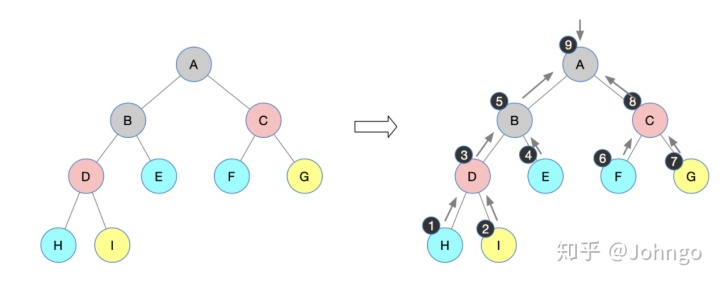按照上述后序遍历的过程，得到后序遍历序列：H I D E B F G C A递归实现二叉树的后序遍历利用上述的递归思想进行C语言代码实现：树形结构按照上述树形结构进行初始化# include <stdio.h>
# include <string.h>
# include <stdlib.h>
# define ElementType char

//结点结构体
typedef struct BinTNode{
ElementType data;
struct BinTNode * left;
struct BinTNode * right;
}BinTNode, *BinTree;

// 初始化树形结构
BinTNode * CreateBiTree(BinTNode *T){
T=(BinTNode*)malloc(sizeof(BinTNode));
T->data='A';
T->left=(BinTNode*)malloc(sizeof(BinTNode));
T->left->data='B';
T->right=(BinTNode*)malloc(sizeof(BinTNode));
T->right->data='C';

T->left->left=(BinTNode*)malloc(sizeof(BinTNode));
T->left->left->data='D';
T->left->right=(BinTNode*)malloc(sizeof(BinTNode));
T->left->right->data='E';
T->left->right->left=NULL;
T->left->right->right=NULL;
T->left->left->left=(BinTNode*)malloc(sizeof(BinTNode));
T->left->left->left->data='H';
T->left->left->left->left=NULL;
T->left->left->left->right=NULL;
T->left->left->right=(BinTNode*)malloc(sizeof(BinTNode));
T->left->left->right->data='I';
T->left->left->right->left=NULL;
T->left->left->right->right=NULL;

T->right->left=(BinTNode*)malloc(sizeof(BinTNode));
T->right->left->data='F';
T->right->left->left=NULL;
T->right->left->right=NULL;
T->right->right=(BinTNode*)malloc(sizeof(BinTNode));
T->right->right->data='G';
T->right->right->left=NULL;
T->right->right->right=NULL;

return T;
}

// 遍历过程中，输出节点值
void printElement(BinTNode * T){
printf("%c ",T->data);
}

//先序遍历
void PostOrderTraverse(BinTNode * T){
if (T) {
PostOrderTraverse(T->left);  //递归访问左孩子
PostOrderTraverse(T->right); //递归访问右孩子
printElement(T);             //输出节点值
}
// 当节点为空的时候，返回
return;
}

int main() {
BinTNode * Tree;
Tree = CreateBiTree(Tree);	// 初始化树形结构
printf("后序遍历: ");
PostOrderTraverse(Tree); 	// 先序递归进行
printf("n");   		// 最后换行
return 0;
}运行结果：后序遍历: H I D E B F G C A非递归实现相比于之前的先序遍历和中序遍历非递归实现，后序遍历的非递归算法与之前有所不同，后续遍历需要先访问左右子结点后，才能访问该结点，而这也是非递归的难点所在。考虑了几种实现的方案，这里我给出我认为比较清晰的一个方案供大家参考：借助两个栈（S1和S2）来进行操作看下面伪代码：初始化伪代码可能看了之后有些不太好懂，但是还算看着清晰吧，下面借助图，一定会思路清晰的（长图发放）：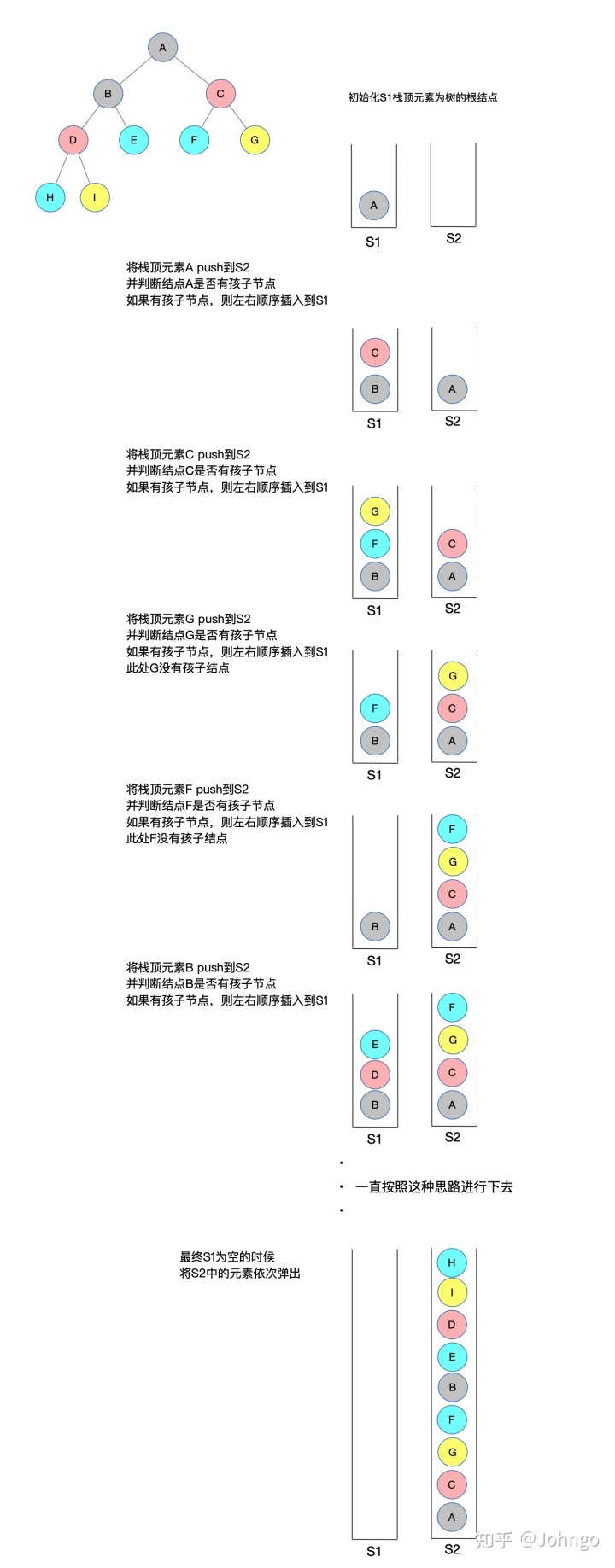有了这个思路就应该会很清晰了，下面按照上述思路用C语言实现：#include <stdio.h>
#include <string.h>
#include <stdlib.h>
#define ElementType char
int top_S1 = -1;   //定义栈S1 top下标
int top_S2 = -1;   //定义栈S2 top下标

// 结点结构体
typedef struct BinTNode{
ElementType data;
struct BinTNode * left;
struct BinTNode * right;
}BinTNode, *BinTree;

// 初始化树形结构
BinTNode * CreateBiTree(BinTNode *T) {
T=(BinTNode*)malloc(sizeof(BinTNode));
T->data='A';
T->left=(BinTNode*)malloc(sizeof(BinTNode));
T->left->data='B';
T->right=(BinTNode*)malloc(sizeof(BinTNode));
T->right->data='C';

T->left->left=(BinTNode*)malloc(sizeof(BinTNode));
T->left->left->data='D';
T->left->right=(BinTNode*)malloc(sizeof(BinTNode));
T->left->right->data='E';
T->left->right->left=NULL;
T->left->right->right=NULL;
T->left->left->left=(BinTNode*)malloc(sizeof(BinTNode));
T->left->left->left->data='H';
T->left->left->left->left=NULL;
T->left->left->left->right=NULL;
T->left->left->right=(BinTNode*)malloc(sizeof(BinTNode));
T->left->left->right->data='I';
T->left->left->right->left=NULL;
T->left->left->right->right=NULL;

T->right->left=(BinTNode*)malloc(sizeof(BinTNode));
T->right->left->data='F';
T->right->left->left=NULL;
T->right->left->right=NULL;
T->right->right=(BinTNode*)malloc(sizeof(BinTNode));
T->right->right->data='G';
T->right->right->left=NULL;
T->right->right->right=NULL;

return T;
}

// 栈S1 - 进栈push
void push_S1(BinTNode** stack,BinTNode * elem) {
stack[++top_S1] = elem;
}

// 栈S2 - 进栈push
void push_S2(BinTNode** stack,BinTNode * elem) {
stack[++top_S2] = elem;
}

//栈S1 - 弹栈pop
void pop_S1(){
if (top_S1 == -1) {
return ;
}
top_S1--;
}

//栈S2 - 弹栈pop
void pop_S2(){
if (top_S2 == -1) {
return ;
}
top_S2--;
}

// 遍历过程中，输出结点值
void printElement(BinTNode* elem) {
printf("%c ",elem->data);
}

//获取栈顶元素
BinTNode * getTop_S1(BinTNode** stack){
return stack[top_S1];
}

//获取栈顶元素
BinTNode * getTop_S2(BinTNode** stack){
return stack[top_S2];
}

//非递归遍历 - 左右根
void PostOrderTraverse(BinTNode * Tree) {
BinTNode * S1;          // 辅助栈
BinTNode * S2;
BinTNode * T = Tree;        // 定义临时指针
BinTNode * Temp;
push_S1(S1, T);             // 初始化S1的top元素是树的根结点
while(top_S1 != -1) {
T = getTop_S1(S1);      // S1的栈顶元素弹出
pop_S1();               // 栈顶元素弹栈
push_S2(S2, T);

if(T->left != NULL || T->right != NULL) {  // 栈顶元素有孩子结点
if(T->left != NULL)
push_S1(S1, T->left);
if(T->right != NULL)
push_S1(S1, T->right);
}
}
// 逐个弹出S2的元素
while(top_S2 != -1) {
printElement(getTop_S2(S2));
pop_S2();
}
}

int main() {
BinTNode * Tree;
Tree = CreateBiTree(Tree);
printf("看到这样就对了: H I D E B F G C An");
printf("后序遍历:t");
PostOrderTraverse(Tree);
printf("n");
return 0;
}运行结果看到这样就对了: H D I B E A F C G
中序遍历:      H D I B E A F C G     DP问题，欢迎关注    动态规划一篇就够了 全网最详细, 逐步理解, 万字总结 - Johngo的文章 - 知乎 https://zhuanlan.zhihu.com/p/130743652后续会将更多的数据结构用C语言代码实现，欢迎大家关注!作者：Johngo计算广告，广告收入占到互联网收入的80%以上，一起研究流量变现，欢迎大家的加入
展开全文• 二叉树的非递归前、中、后序遍历算法详解及代码实现...2. 后序遍历非递归算法思路 后序非递归遍历的C代码 1. 前序遍历和中序遍历非递归算法思路 遍历过程：（如图所示） 图1 前序遍历和中序遍历流程图   ...

转载请注明出处：https://blog.csdn.net/Benja_K/article/details/88389039
二叉树的非递归前、中、后序遍历算法详解及代码实现（C语言）
1. 前序遍历和中序遍历非递归算法思路
前序和中序非递归遍历的C代码
2. 后序遍历非递归算法思路
后序非递归遍历的C代码
1. 前序遍历和中序遍历非递归算法思路
遍历过程：（如图所示）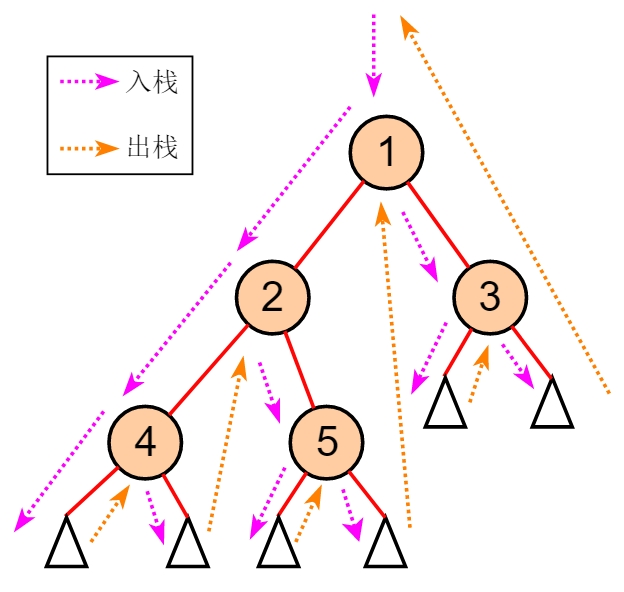图1 前序遍历和中序遍历流程图

从当前节点开始遍历：（当入栈时访问节点内容，则为前序遍历；出栈时访问，则为中序遍历）
1. 若当前节点存在，就存入栈中，并访问左子树；
2. 直到当前节点不存在，就出栈，并通过栈顶节点访问右子树；
3. 不断重复12，直到当前节点不存在且栈空。

Tips：我们很容易发现，在理解并写好该非递归遍历代码后，只需要在入栈、出栈的时候，分别进行节点访问输出，即可分别得到前序、中序的非递归遍历代码！！！

便于理解的伪代码：
void preOrder(TreeNode *T){    p = T    while(p不空||栈不空){        if(p不空){      //两种情况：1.栈不空；2.栈空            p入栈;            (前序遍历，访问)            入p左子节点;        }else{            //一种情况：当前节点为空，但栈不空            p=出栈;            (中序遍历，访问)            入p右子节点;        }    }}
前序和中序非递归遍历的C代码
二叉树节点结构体：
typedef struct TreeNode{    int data;    struct TreeNode *lChild;    struct TreeNode *rChild;}TreeNode;
前序遍历非递归：
void preOrder(TreeNode *T){    TreeNode *stack;    int top = -1;    TreeNode *p = T;    while(p!=NULL||top!=-1){        if(p!=NULL){            stack[++ top] = p;            printf("%d\t",p->data); //入栈时，访问输出            p = p->lChild;        }else{            p = stack[top --];            p = p->rChild;        }    }}
中序遍历非递归：
void inOrder(TreeNode *T){    TreeNode *stack;    int top = -1;    TreeNode *p = T;    while(p!=NULL||top!=-1){        if(p!=NULL){            stack[++ top] = p;            p = p->lChild;        }else{            p = stack[top --];            printf("%d\t",p->data);  //出栈时，访问输出            p = p->rChild;        }    }}
2. 后序遍历非递归算法思路
后序遍历整体与前中序遍历过程相似。但要注意，这时对于父节点的访问输出，需要在其右子树遍历完成的前提下进行。所以不能像前中序遍历一样，在遍历完左子树后，就直接出栈。我们需要利用这个未出栈的栈顶元素去获取右子树，在遍历完右子树后，就可以出栈，并对此节点进行访问输出。
这里我们需要使用一个标记，以区分是从左子树取栈还是从右子树出栈：（如图所示）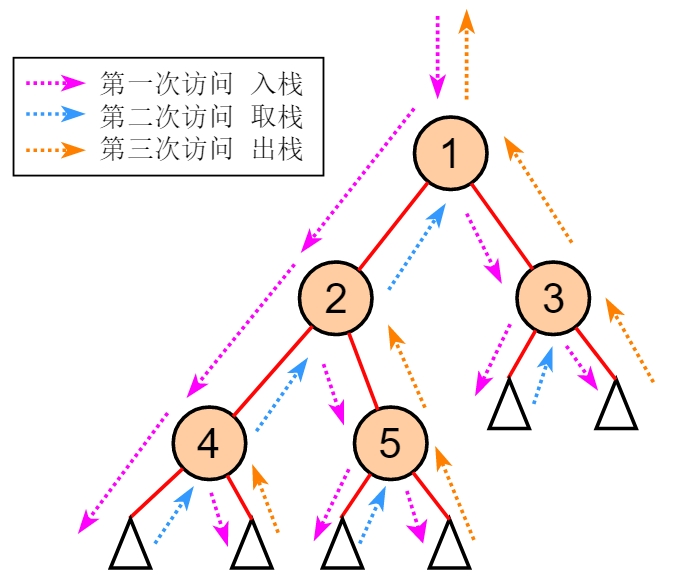图2 后序遍历流程图

从当前节点开始遍历：
1. 若当前节点存在，就存入栈中，并且置节点flag为1（第一次访问），然后访问其左子树；
2. 直到当前节点不存在，需要回退，这里有两种情况：
1）当栈顶节点flag为1时，则表明是从左子树回退，这时需置栈顶节点flag为2（第二次访问），然后通过栈顶节点访问其右子树（取栈顶节点用，但不出栈）
2）当栈顶节点flag为2时，则表明是从右子树回退，这时需出栈，并取出栈节点做访问输出。（需要注意的是，输出完毕需要置当前节点为空，以便继续回退。具体可参考代码中的p = NULL）
3. 不断重复12，直到当前节点不存在且栈空。

Tips：我们很容易发现，当理解并写好后序遍历非递归代码后，只需要在入栈、取栈、出栈的时候，分别进行节点访问输出，即可分别得到前序、中序、后序的非递归遍历代码，是不是非常简单！！！

后序非递归遍历的C代码
节点结构体：
typedef struct TreeNode{    int data;    struct TreeNode *lChild;    struct TreeNode *rChild;}TreeNode;
后序遍历非递归：
void postOrder(TreeNode *T){    TreeNode *stack;    int top = -1;    int flagStack;   //记录每个节点访问次数栈    TreeNode *p = T;    while(p!=NULL||top!=-1){        if(p!=NULL){     //第一次访问，flag置1，入栈            stack[++ top] = p;            flagStack[top] = 1;               p = p->lChild;        }else{//（p == NULL）            if(flagStack[top] == 1){  //第二次访问，flag置2，取栈顶元素但不出栈                p = stack[top];                flagStack[top] = 2;                p = p->rChild;            }else{         //第三次访问，出栈                p = stack[top --];                printf("%d\t",p->data);    //出栈时，访问输出                p = NULL;      //p置空，以便继续退栈            }        }    }}


展开全文数据结构
•   一直说要写二叉树的后序非递归遍历算法，但是前两天各种事情，今天终于有时间好好写一写二叉树后序遍历算法。   二叉树后序遍历算法比先序和中序的遍历算法要复杂一些。其出栈条件有两种情况： 栈顶元素...数据结构
• 首先非常感谢‘hicjiajia’的博文：二叉树后序遍历(非递归) 这篇随笔开启我的博客进程，成为万千程序员中的一员，坚持走到更远！ 折磨了我一下午的后序遍历中午得到解决，关键在于标记右子树是否被访问过，考虑过...
• ---使用非递归实现二、解题思路非递归后序遍历 --- 左右根对于一个节点而言，要实现访问顺序为左儿子-右儿子-根节点，可以利用后进先出的栈，在节点不为空的前提下，依次将根，右，左压栈。故需要按照根-右-左的顺序...
• 后序遍历 后序遍历要求左右子树均完成后才对自身进行访问。入栈时，先将右子树（若存在）入栈再将左子树（若存在）入栈，然后指向左孩子（若存在左孩子，否则指向右孩子）。在对这个部分结点构成的栈进行出栈操作时...
• 数据结构二叉树遍历基础，递归解法在很早之前的博客就以C语言的形式总结了，这篇博文聚焦非递归解法。二叉树的前序、中序、后序遍历都可以借助栈来实现，因为递归本质也是靠栈来实现的，中序遍历还有一种比较难想的...数据结构 算法
• 二叉树先序遍历的实现思想是：访问根节点；访问当前节点的左子树；若当前节点无左子树，则访问当前节点的右子树；图 1 二叉树以图 1 为例，采用先序遍历的思想遍历二叉树的过程为：访问该二叉树的根节点，找到 1；...
• int* postorderTraversal(struct TreeNode* root, int* returnSize){ int *ret=(int*)malloc(sizeof(int)*100); struct TreeNode** stack=(struct TreeNode**)malloc(sizeof(struct TreeNode*)*100);...
• 然后非递归的算法这么写的： [img=https://img-bbs.csdn.net/upload/201511/20/1448026860_114445.png][/img] 但是代码执行时进入130行的死循环了 第134行设置了true之后，在IsChildsAllPrintOut()函数里获取的...
• 1.实验目的 熟练掌握二叉树的二叉链表存储结构的C语言实现。...（2）二叉树的前、中、后序遍历的递归算法和非递归算法的实现 （3）求二叉树中叶子结点数目的递归算法。 （4）编写求二叉树深度的递归算法。 ...C
• typedef struct BiTNode //二叉树节点 { int data; struct BiTNode *lchild, *rchild; }BiTNode, *BiTree; 定义函数： void InitTree(BiTree &); //初始化 void Visit(BiTree); //访问节点数据 void PreOrder...算法 数据结构 c++
• 145. 二叉树后序遍历非递归）1. 题目描述2.代码如下3.细节 1. 题目描述 给定一个二叉树，返回它的 后序 遍历。 示例: 输入: [1,null,2,3] 1 2 / 3 输出: [3,2,1] 进阶: 递归算法很简单，你可以通过迭代算法完成...
• 题目 代码 int* postorderTraversal(struct TreeNode* root, int* returnSize){ *returnSize = 0; if (root == NULL) return 0; #define MAX 1000000 int *arr = (int *)malloc(sizeof(int) * MAX);...
• 二叉树 非递归前中后序遍历汇总 C语言 希望大家给予建议
• 欢迎大家交流指正 #include<stdio.h> #include<stdlib.h> #define MAXSIZE_QUEUE 1000 #define MAXSIZE_STACK 1000 typedef char TElemType;... //非递归后续遍历使用,用于统计次数数据结构
• 1.输入前序和中序遍历结果，建立二叉树 2.实现二叉树的三种递归遍历算方法 3.实现二叉树的三种非递归遍历算法 4.实现二叉树的旋转90°后的打印，直观树形结构
• 二叉树的非递归前、中、后序遍历算法详解及代码实现（C语言） ...2. 后序遍历非递归算法思路 后序非递归遍历的C代码 1. 前序遍历和中序遍历非递归算法思路 遍历过程：（如图所示） 图1 前...数据结构
• 经过两天的搜索，看到网上很多种解法，很多解法都是用C++来写的算法，一直找不到用C语言写的算法，所以就总结了一下，用C写出了一个遍历二叉树的三种非递归算法。 前序遍历 前序遍历按照“根结点-左孩子-右孩子”...数据结构
• 2. 后序遍历非递归算法思路 后序非递归遍历的C代码 1. 前序遍历和中序遍历非递归算法思路 遍历过程：（如图所示） 图1 前序遍历和中序遍历流程图 从当前节点开始遍历：（当入栈时访问节点内容，则为前序......

# 二叉树后序遍历非递归c语言c语言 订阅